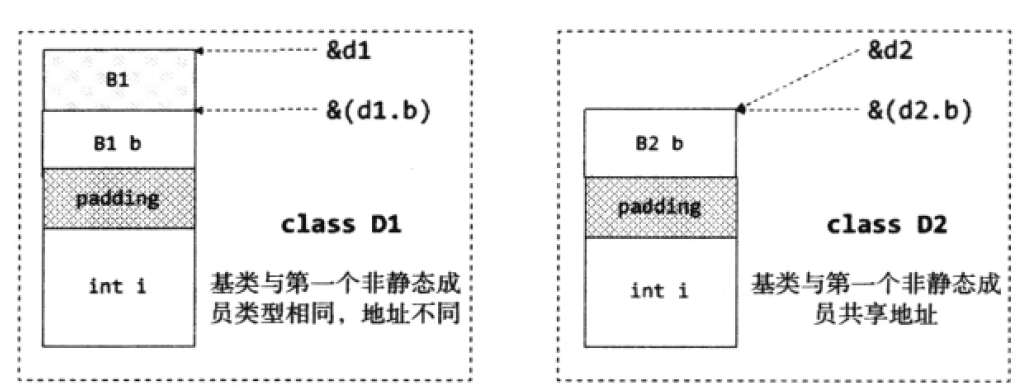# C++11 POD 类型

POD（Plain Old Data，普通旧数据）类型是从 C++11 开始引入的概念，Plain 代表一个对象是一个普通类型，Old 代表一个对象可以与 C 兼容。通俗地讲，一个类、结构、共用体对象或非构造类型对象能通过二进制拷贝（如 memcpy()）后还能保持其数据不变正常使用的就是POD类型的对象。严格来讲，一个对象既是普通类型（Trivial Type）又是标准布局类型（Standard-layout Type）那么这个对象就是 POD 类型。

C++ 给定对象类型取决于其特定的内存布局方式，一个对象是普通、标准布局还是 POD 类型，可以根据标准库函数模板来判断：

is_trivial<T>
is_standard_layout<T>
is_pod<T>


# 1.普通类型

（1）没有虚函数或虚基类；
（2）由C++编译器提供默认的特殊成员函数（默认的构造函数、拷贝构造函数、移动构造函数、赋值运算符、移动赋值运算符和析构函数）；
（3）数据成员同样需要满足条件（1）和（2）。

#include <iostream>
#include <string>

class A { A() {} };
class B { B(B&) {} };
class C { C(C&&) {} };
class D { D operator=(D&) {} };
class E { E operator=(E&&) {} };
class F { ~F() {} };
class G { virtual void foo() = 0; };
class H : virtual F{};
class I {};

int main()
{
std::cout << std::is_trivial<A>::value ;  //有自定义构造函数
std::cout << std::is_trivial<B>::value;  //有自定义的拷贝构造函数
std::cout << std::is_trivial<C>::value;  //有自定义的移动构造运算符
std::cout << std::is_trivial<D>::value;  //有自定义的赋值运算符
std::cout << std::is_trivial<E>::value;  //有自定义的移动赋值运算符
std::cout << std::is_trivial<F>::value;  //有自定义的析构函数
std::cout << std::is_trivial<G>::value;  //有虚函数
std::cout << std::is_trivial<H>::value;  //有虚基类
std::cout << std::is_trivial<I>::value ;   //普通的类return 0;
}


000000001


# 2.标准布局类型

（1）没有虚函数或虚基类；
（2）所有非静态数据成员都具有相同的访问说明符；
（3）在继承体系中最多只有一个类中有非静态数据成员；
（4）子类中的第一个非静态成员的类型与其基类不同；此规则是因为 C++ 允许优化不包含成员基类而产生的。在 C++ 标准中，如果基类没有任何数据成员，基类应不占用空间，为了体现这一点，C++ 标准允许派生类的第一个成员与基类共享同一地址空间。但是如果派生类的第一个非静态成员的类型和基类相同，由于 C++ 标准要求相同类型的对象的地址必须不同，编译器就会为基类分派一个字节的地址空间。比如下面的代码：

class B1{};
class B2{};

class D1: public B1
{
B1 b;
int i ;
};
class D2: public B1
{
B2 b ;
int i ;
}


D1 和 D2 类型的对象内存布局应该是相同的，但实际上是不同的，因为 D1 中基类 B1 和对象 b 都占用了 1 个字节，D2 中基类 B1 为空，并不占用内存空间。D1 和 D2 的内容布局从左至右如下图所示：（5）所有非静态数据成员同样需要满足条件（1）、（2）、（3）和（4）。

#include <iostream>
using namespace std;

class A { virtual void foo() = 0; };

class B
{
private:
int a;
public:
int b;
};

class C1
{
int x1;
};

class C:C1
{
int x;
};

class D1 {};
class D : D1
{
D1 d1;
};

class E : virtual C1 {};
class F { B x; };
class G :C1, D1 {};

int main()
{
std::cout << std::is_standard_layout<A>::value ;  // 有虚函数
std::cout << std::is_standard_layout<B>::value ;  // 成员a和b具有不同的访问权限
std::cout << std::is_standard_layout<C>::value ;  // 继承树有非静态数据成员的类超过1个
std::cout << std::is_standard_layout<D>::value ;  // 第一个非静态成员是基类类型
std::cout << std::is_standard_layout<E>::value ;  // 有虚基类
std::cout << std::is_standard_layout<F>::value ;  // 非静态成员x不符合标准布局类型
std::cout << std::is_standard_layout<G>::value ;  // return 1
return 0;
}


00000001


# 3.POD 类型简介

（1）可以使用字节赋值，比如用 memset、memcpy 对 POD 类型进行赋值操作；
（2）对 C 内存布局兼容，POD 类型的数据可以使用 C 函数进行操作且总是安全的；
（3）保证了静态初始化的安全有效，静态初始化可以提高性能，如将 POD 类型对象放入 BSS 段默认初始化为 0。

#include <iostream>
using namespace std;

class A
{
public:
int x;
double y;
};

int main()
{
if (std::is_pod<A>::value)
{
std::cout << "before" << std::endl;
A a;
a.x = 8;
a.y = 10.5;
std::cout << a.x << std::endl;
std::cout << a.y << std::endl;

size_t size = sizeof(a);
char *p = new char[size];
memcpy(p, &a, size);
A *pA = (A*)p;

std::cout << "after" << std::endl;
std::cout << pA->x << std::endl;
std::cout << pA->y << std::endl;

delete p;
}
return 0;
}


before
8
10.5
after
8
10.5


# 参考文献

 Trivial、standard-layout 和 POD 类型
 C++11之POD类型
 C++11新特性之POD类型
 POD (程序设计).wikipedia
 深入理解C++11[M].C3.8POD类型

03-198466

#### C++中POD是什么？06-296638

#### C++11：POD数据类型

06-241480

#### 《深入理解C++11》笔记–POD类型

10-238932

#### c++的POD类型详解

06-075265

#### C++之POD数据类型

05-311694

#### C++11新特性之POD类型

03-12339

#### 非POD类型

04-13157

#### [STL源码剖析]POD类型©️2020 CSDN 皮肤主题: 编程工作室 设计师: CSDN官方博客点击重新获取扫码支付1.余额是钱包充值的虚拟货币，按照1:1的比例进行支付金额的抵扣。
2.余额无法直接购买下载，可以购买VIP、C币套餐、付费专栏及课程。余额充值